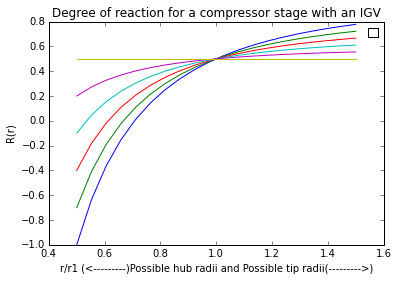# Chapter7-Axial Compressor Aerodynamics¶

## Ex1-pg397¶

In :
import math
#calculate specific work at pitch line and rotor torque per unit mass flow rate
w=5600. ##rpm
rm=0.5 ##m
Ct2=145. ##m/s
Um=w*2*math.pi*rm/60. ##Rotor tangential speed at pitchline in m/s
Ct1=0.
dU=Ct2-Ct1
wc=Um*dU/1000. ## in kJ/kg
tpm=rm*(dU)
print'%s %.6f %s'%("Specific work at pitchline in",wc, "kJ/kg")
print'%s %.1f %s'%("Rotor torque per unit mass flow rate in ",tpm,"m^2/s")

Specific work at pitchline in 42.516221 kJ/kg
Rotor torque per unit mass flow rate in  72.5 m^2/s


## Ex2-pg407¶

In :
import math
#calculate rotor anugular speed and rotor exit swirl and rotor specific work at pitch line and rotor mass flow rate and stotor mass flow rate and flow efficient
rm=0.5
Um=212. ##m/s
Czm=155. ##m/s
Ct1m=28. ##m/s
Rm=0.6
alfar=1. ##alfar=alfa3/alfa1.
w=Um*60./(rm*2*math.pi)
print'%s %.f %s'%("Rotor angular speed w in",w," rpm")
Ct2m=2.*Um*(1.-Rm)-Ct1m
print'%s %.f %s'%("Rotor exit swirl in",Ct2m," m/s")
wcm=Um*(Ct2m-Ct1m)/1000.
print'%s %.f %s'%("Rotor specific work at pitchline Wcm in",wcm," kJ/kg ")
Wt2m=Ct2m-Um
print'%s %.f %s'%("Rotor relative velocity vector at rotor exit in",Wt2m," m/s")
print("Hence vector is 155k-70.4e")
##Since alfa3=alfa1, rotor and stator torques are equal and opposite each other.
trm=rm*(Ct2m-Ct1m)
tsm=-1*trm
print'%s %.f %s'%("Rotor torque per unit mass flow rate in",trm," m^2/s")
print'%s %.f %s'%("stotor torque per unit mass flow rate in",tsm," m^2/s")
pshm=(Ct2m-Ct1m)/Um
phm=Czm/Um
print'%s %.f %s'%("Flow coefficient",phm,"")

Rotor angular speed w in 4049  rpm
Rotor exit swirl in 142  m/s
Rotor specific work at pitchline Wcm in 24  kJ/kg
Rotor relative velocity vector at rotor exit in -70  m/s
Hence vector is 155k-70.4e
Rotor torque per unit mass flow rate in 57  m^2/s
stotor torque per unit mass flow rate in -57  m^2/s
Flow coefficient 1


## Ex3-pg409¶

In :
import math
#determine circulation and D-factor
Um1=200. ## in m/s
Um2=Um1
Cz1=150. ##in m/s
Cz2=Cz1
b2=-35. ##in degree
Cm=7. ##in cm
Sm=7. ##in cm
W1m=((Um1**2.)+Cz1**2.)**(1/2.)
Wt2m=Cz2*math.tan(-35/57.3)
W2m=((Cz1)**2.+(Wt2m)**2.)**(1/2.)
print"%s %.1f %s"%("W1m in ",W1m,"m/s:")
print"%s %.4f %s"%("W2m in ",W2m,"m/s ")
sigma=Cm/Sm
Wt1m=-1.*Um1
Dm=1.-(W2m/W1m)+(abs(Wt2m-Wt1m))/(2.*sigma*W1m)
print"%s %.4f %s"%("D-factor Dm",Dm," ")
Tm=Sm/100.*abs(Wt1m-Wt2m)
print"%s %.7f %s"%("Circulation Tm in ",Tm,"m^2/s")

W1m in  250.0 m/s:
W2m in  183.1104 m/s
D-factor Dm 0.4575
Circulation Tm in  6.6485249 m^2/s


## Ex4-pg421¶

In :
import math
#calculate static pressure rise co-efficent
W1=300. ##in m/s
wrm=0.03
W2min=0.72*W1
Cp=1-(W2min/W1)**2-wrm
print"%s %.1f %s"%("Minimum W2 in",W2min," m/s :")
print"%s %.3f %s"%("Static pressure rise coefficient",Cp,"")

Minimum W2 in 216.0  m/s :
Static pressure rise coefficient 0.452


## Ex5-pg429¶

In :
import math
#calculate total temperature ratio and compressor polytropic efficency
ps=1.5
es=0.9
gm=1.4
ts=1.+(1./es)*(ps**((gm-1.)/gm)-1.)
ec=(gm-1.)/gm*(math.log(ps))/math.log(ts)
print"%s %.3f %s"%("Total temperature ratio",ts,"")
print"%s %.3f %s"%("Compressor polytropic efficiency",ec,"")

Total temperature ratio 1.136
Compressor polytropic efficiency 0.906


## Ex6-pg429¶

In :
import math
#calculate mean relative flow angle and rotor section drag co-efficent and rotor circulation and rotor sectional 2d lift co-efficent
W1=460. ##in m/s
b1=45.##degrees
W2=376.
b2=30.
c=5.25
w=0.05
s=3.5
Wt1=W1*math.sin(45/57.3)
Wt2=W2*math.sin(30/57.3)
Wtm=(Wt1+Wt2)/2
Wz1=W1*math.cos(45/57.3)
Wz2=W2*math.cos(30/57.3)
Wz=(Wz1+Wz2)/2
bm=(math.atan(Wtm/Wz))*180/math.pi
sigma=c/s
Cd=w/sigma*math.cos(bm/57.3)
T=s/100*(abs(Wt1-Wt2))
Wm=(Wz**2+Wtm**2)**(1/2.)
C1=2.*T/(Wm*(c/100.))-Cd*math.tan(bm/57.3)
print"%s %.4f %s"%("mean relative flow angle :",bm,"")
print"%s %.4f %s"%("The rotor section (2D) drag coefficient :",Cd,"")
print"%s %.4f %s"%("The rotor circulation in m**2/s :",T,"")
print"%s %.4f %s"%("The rotor sectional (2D) lift coefficient :",C1,"")

mean relative flow angle : 38.2551
The rotor section (2D) drag coefficient : 0.0262
The rotor circulation in m**2/s : 4.8042
The rotor sectional (2D) lift coefficient : 0.4209


## Ex7-pg437¶

In :
print "Example 7.7"
%matplotlib inline
import warnings
warnings.filterwarnings('ignore')
#calculate and draw the garph of degree of reaction for compressor stage with IGV and possible hub radii and possible tip radii
import numpy
import matplotlib
from matplotlib import pyplot
Rm=0.5
b=0 #b=b/w
i=1
z0=numpy.linspace(0.,.5,6)
z1=numpy.linspace(0.5,1.5,20)
for b in z0:
r=0.5
vr=z1;
x=numpy.zeros(20)
count=0;
for r in z1:
R=(1-b)-((1-b)-Rm)/(r)**2
x[count]=R
count=count+1;
pyplot.plot(vr,x)
i=i+1;

Example 7.7